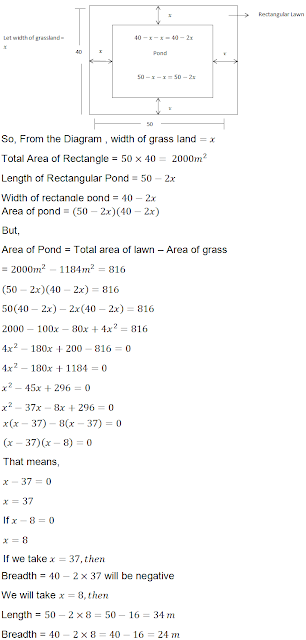>

## Chapter 8 Quadratic Equations R.D. Sharma Solutions for Class 10th Math Exercise 8.11

1. The perimeter of a rectangular field is 82 m and its area is 400 m2. Find the breadth of the rectangle.

Solution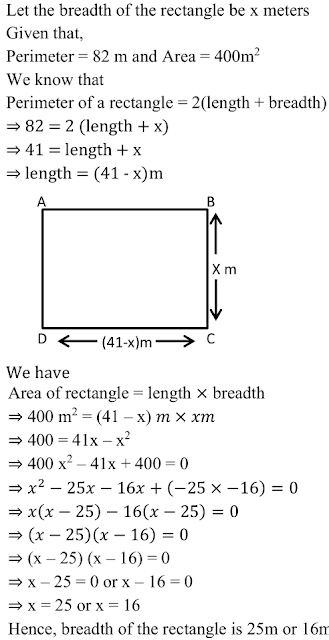2. The length of a hail is 5 m more than its breadth. If the area of the floor of the hail is 84 m2 , what are the length and breadth of the hail ?

Solution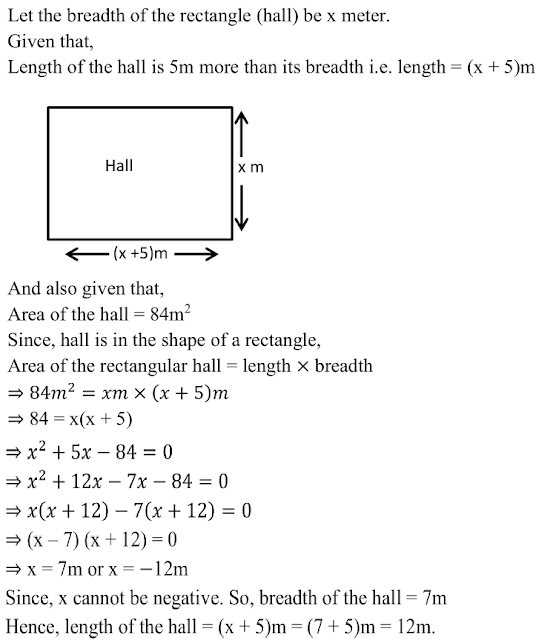3. Two squares have sides x cm and (x + 4) cm. The sum of their areas is 656 cm2 . Find the sides of the squares.

Solution4. The area of a right angled triangle is 165 m2. Determine its base and altitude if the latter exceeds the former by 7m.

Solution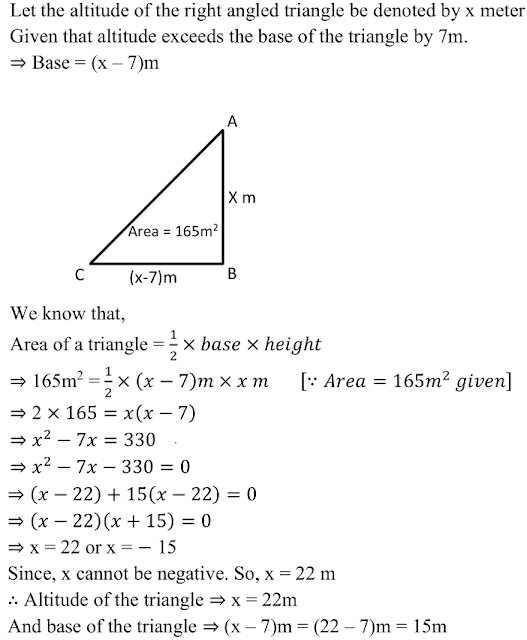5. Is it possible to design a rectangular mango grove whose length is twice its breadth and the area is 800 m2? If so, find its length and breadth.

Solution6. Is it possible to design a rectangular park of perimeter 80 m and area 400 m2? If so, find its length and breadth.

Solution7. Sum of the areas of two squares is 640 2. If the difference of their perimeters is 64 m, find the sides of the two squares.

Solution8. sum of the areas of two squares is 400 c2. If the differences of their perimeters is 16 cm, find the sides of two squares.

Solution9. The area of a rectangle plot is 528 cm2. The length of the plot (in metres) is one metre more then twice its breadth . Find the length and the breadth of the plot .

Solution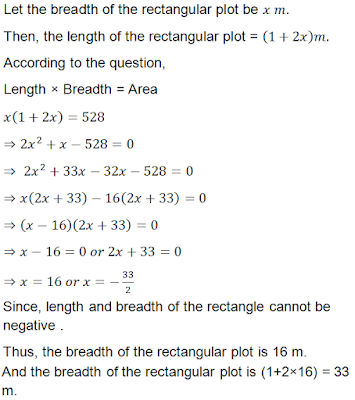10. In the centre of a rectangular lawn of dimensions 50 m × 40 m , a rectangular pond has to be constructed so that the area of the grass surrounding the pond would be 1184 2. Find the length and breadth of the pond .

Solution